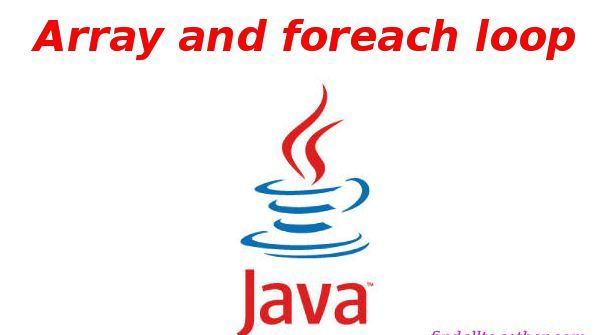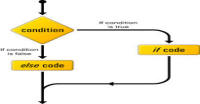# Array in java

Array is variable which is a collection of variables of same data types. You can assume arrays as blocks placed in a row in which you can fill your data with a restriction that all data must be of same type. We can define an array in three ways:

```package flowkl;

public class Tutorials
{
public static void main(String args[])
{
// syntax of array
// array of integers
// first method of initialisation
int array1[] = {2,3,4};

// second method
// define array
int[] array2 = new int;;
// above line is equivalent to int array2[];
// collection of 3 variables but still all values are uninitialised

// initialise values
array2 = 2;
array2 = 3;
array2 = 4;

// third method
int array3[];
// above array (array2) is null or uninitialised
array3 = new int;

// initialise values
array3 = 2;
array3 = 3;
array3 = 4;

}
}
```

Note these points:

1. array1 is collection of three integer variables => array1, array and array. We access these variables using there indexes (positions, starting from 0). We have defined all three arrays (array1, array2 and array3 ) as integer but arrays can be any data type like char, double, object etc.
2. Like variables we can define array at one place and initialize it at another place (like array2 and array3). It is useful property because in practical programs we use mostly this type of initialisations.

### Array with loop:

We access array with loop like this:

```package flowkl;

public class Tutorials
{
public static void main(String args[])
{
// Initialise array
double list[] = {2.13 ,3 ,4.4 ,64.54 ,75};

// for loop
// length is property of array and returns value of
// number of elements in the array, here 5
for(int i = 0;i< list.length;i++)
{
// here we have each element of array one by one
System.out.println(list[i]);
}
}
}
```

### Foreach loop in java:

Now we can use foreach loop to access each element of array inside the loop one by one. In foreach loop, we get next element of the array inside the loop. This example is equivalent to above example:

```package flowkl;

public class Tutorials
{
public static void main(String args[])
{
// Initialise array
double list[] = {2.13,3,4.4,64.54,75};

// for loop
for(double temp : list)
{
// here we have each element of array one by one
// we are assigning next element of list to temp in each iteration
// starting from first element
System.out.println(temp);
}
}
}
```

Note that data type of temporary variable ( temp ) must be same as iterative variable ( list ).

### Multidimensional Array:

Notice that an array is a variable and it contains variables. So what will happen if we make an array of type array? It will be a multidimensional array. For a two dimensional array you may assume it as a table of m*n. For example, I am storing following data in a two dimensional array:

 12 17 28 83 23 98 9 83 32 90 92 31

The code will be:

```package flowkl;

public class Tutorials
{
public static void main(String args[])
{
/*
* table is :
* 12, 17, 28
* 83, 23, 98
* 9, 83, 32
* 90, 92, 31
*/
// it is (array[])[] i.e. array of arrays
// equivalent to int[][] multiarray
int multiarray[][] = {
// each row is a new array
{12, 17, 28},
{83,23,98},
{9,83,32},
{90,92,31}
};

// for loop for multidimensional array
// multiarray is our first array
for(int i = 0;i < multiarray.length;i++)
{
/*
* inside multiarray we have 4 arrays
* multiarray to multiarray
* i is also varying from 0 to 3
* so our inner arrays are multiarray[i]
*/
// in other words we have row of table as multiarray[i]

for(int j = 0; j < multiarray[i].length;j++)
{
/*
* here we have multiarray[i][j]
* if we show in table then we have
* ith row and jth column of the table
*/

// we are printing table
// this will print one row for each inner loop
// or say for each value of i, j will vary from 0 to lenght of row
System.out.print(multiarray[i][j] + " ");
}

//after each row we want to change the line
System.out.println();
}
}
}
```

This example is lengthy and some complex also. If you could not understand this example then don't worry. I am describing it again.

• In line 16, we are defining a multidimensional array. It is defined by placing one more square braces after variable miltiarray. If we place one more square braces after it then ir will be 3-dimensional. We are pacing elements (values) of each row in a new array. So we have 4 inner arrays, each corresponding to each row. Elements of each array are columns.
• In line 26, we are writing a loop for outer array (multiarray). This will navigate to its each element (each row) which are arrays.
• In line 36, we are using one more loop. In this loop, we have one array (row) each time say multiarray[i] (ith row). After inside this loop we have one by one each element (column) of that array (row). We are printing that element (column) in line 47.
• Line 51 is outside the inner loop but still inside the outer for loop. This line will be executed after printing each array (row) and will print a new line (will change line).
• By this way we are printing a table. If we have to operate a 3-dimensional array then we will require 3 loops.

In above example, variable can define and initialize by second and third methods (defined in first example). Multidimensional arrays can also be operated using foreach loops. We can write above example like this:

```package flowkl;

public class Tutorials
{
public static void main(String args[])
{
// 4 rows and 3 columns
// in this case all columns must be of
// equal lenght say 3
int multiarray[][] = new int;

// each row is a new array
multiarray =    12;
multiarray =    17;
multiarray =    28;
multiarray =    83;
multiarray =    23;
multiarray =    98;
multiarray =    90;
multiarray =    92;
multiarray =    31;

// for loop for multidimensional array
// multiarray is our first array
for(int[] outside: multiarray)
{
// each time a new row will be assign to outside

for(int inside : outside)
{
// each column will be assing to inside each time
System.out.print(inside + " ");
}

//after each row we want to change the line
System.out.println();
}
}
}
```

#### Click on banner below to Learn: PHP with Laravel for beginners - Become a Master in LaravelHarish, a technical core team member at www.lyflink.com with five year experience in full stack web and mobile development, spends most of his time on coding, reading, analysing and curiously following businesses environments. He is a non-graduate alumni from IIT Roorkee, Computer Science and frequently writes on both technical and business topics.

### Related ArticlesPHP stands for PHP: Hypertext Preprocessor. PHP is known as a server side scripting and interpreter language for web ...
##### Introduction to PHPIn this tutorial, we will study about conditional statements (if else statements and switch statements). These statem...
##### Conditional statements in phpIn this tutorial, we will study about strings and operations upon strings. Due to long length of this section, I have...

### The Complete Web Developer Course - Build 25 Websites